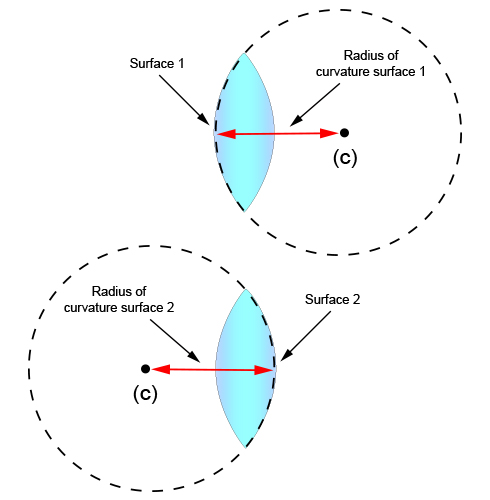# Convex lens: radius of curvature (R)

We should all know what a radius of a sphere is, so it should be easy to remember that:

The "radius of curvature" of a convex lens is the distance between the centre of curvature (C) and the curvature of that sphere.

There are two radius of curvatures for a convex lens, as below: